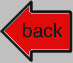# Diffusion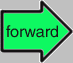## 3. Phenomenological Modelling of Diffusion

Preliminary Remarks"Phenomenological" is a phenomenal word with 7 syllables! A journalist I know was almost fired for using a 5-syllable word in the Ithaca Journal. What does it mean?
According to a dictionary it means "relating to phenomenology", and phenomenology is the "science" of phenomena. That really helps"Phenomenological Modelling" in plain words means that you model what you "see", what you know from experiments. However, you do not just describe some phenomena one by one, you try to find a common underlying pattern. As far as possible, you let yourself be guided by the facts and not by hypotheses or theories about how things should be. If you are lucky, the underlying pattern can be couched in the form of a rule that can be expressed in equations (often and wrongly called a "law") that contains the outcome of all experiments already performed and allows to predict the outcome of experiments that haven't been done yet.Ohm's "law", for example, is probably the best know phenomenological model for certain phenomena concerning the flow of electrical current through some materials. I put the word "law" in quotation marks since Ohm's law was not a law of nature, it just described the essence of many experiments. Today Ohm's law is recognized as a law of nature - but only under certain, precisely defined circumstances. That is so because modern physics can derive Ohm's law from it's basic laws or axioms, and thus knows exactly what is behind it, under what kind of circumstances it is valid, and when and why it might be completely wrong.What we are looking at her is Adolf Fick's first and second "law" of diffusion. You might never have heard about those laws but they are still in the very center of material processing. My students most certainly hear about Fick's laws in detail, and that always involves a certain amount of smirking or giggling because not only is "Adolf" a first name that has not been used for more than 65 year in Germany; Adolf's last name in German means exactly what it would mean in English if you exchange the "i" for another vowel.Adolf Fick, by the way, was not a physicist but a medical Doctor. In 1855 young Fick, employed by the University of Zürich in Switzerland, published a seminal paper in "Poggendorf's Annalen der Physik" (Poggendorf's annals (yearly records) of physics). The title was simply: "Über Diffusion" (About dffusion).How come a medical guy put down some of the more important basic equations of Materials Science? Simple. The diffusion of water through biological membranes like cell walls is a basic process of life. However, it did not attract much attention from the physicists in those times so Fick addressed the matter himself.
For about 50 years Fick's "laws" were empirical and not justified by deeper insights into the mechanisms of diffusion. It was Albert Einstein who derived Fick's law from the atomistic theory of diffusion. In a perfect world that would have been his second Nobel price (with about four more to come). In the world we live in, he only got one.So in what follows I will first work through Ficks's two laws and then tie them to the atomistic modelling of diffusion.

Fick's First and Second LawFirst we need to define the term particle current density. That is actually quite easy. It is just another word for how much of something expressed as number of particles flows through some area in a given time. Since everything that exists can be broken down to particles, we can express any current, anything that flows from here to there, in terms of particles flowing from here to there.Let's look at some examples:
• Water flows through a 3/4'' pipe. What flows on the particle level are the water molecules (H2O). The area Aw it flows through is the cross-sectional area of the pipe; Aw = (p/4) · (3/4'')2 = 0.442 sq.in. = 285 mm2. The water particle current density then is:
No. of water molecules flowing through the area Aw per second.
Of course, you just as well could give the current density in gallons or fluid ounces per second and cross-sectional area since we I know how many molecules one gallon of water contains.
Expressing current densities in numbers of particles simply is more fundamental and international because you don't have to know about monstrosities like inch, gallon, fluid ounces, and so on. Even Americans count 1, 2, 3,... and thus come up with the same number for some particles as you and me (as long as it doesn't involve billions/ trillions). The unit of a particle current density thus is always: No. particles/(s · mm2) = s–1 · mm–2 since "particle number" has no unit.
• Compressed air flows through a 10 mm diameter hose. What flows on the particle level are the oxygen and nitrogen molecules ...
• A cold air draft flows through that door that your daughter never closes. You certainly feel it - but how large is the air particle (essentially oxygen and nitrogen molecule) current density? The cold air flows through the area left open, but there seems to be more cold air coming in around the bottom of the door than on top. In other words: the current flowing through some imagined area (called area increment) at the bottom is larger than at the top, or the current density is a function of position.
Well, that's how it is in most case. So measure the flow through small areas and get the current as function of position or express it as an average for the total cross-section if you like.
• Bright sunlight flows through the window. The particles flowing are photons, the "light particles". Divide your window into little areas or pixels, measure the current density for each pixel, and sum up to obtain the total.
• Electrical current flows through a wire to your TV set. Even that is a particle flow. What you have now is a current density of charged particles and that we call an electrical current density. In your wire those particles are electrons, carrying one negative elementary charge of e = –1.602 · 10–19 C (for "Coulomb") with them. In your battery the charged particles flowing from anode to cathode or the other way around are ions (charged atoms), carrying one or a few negative or positive elementary charges.
A rate of 6.24 · 1018 elementary charges per second we call an (electrical) current of 1 Coulomb per second = 1 A (Ampère). Divide by the cross-sectional area of the wire and you have the (electrical) current density.
• People flowing through some open door from one room to another one during a party. You have no problem counting how many particles = people come through the cross-section given by the door every second. But you do have a problem deciding if you count the ones going from the living room into the dining room, or the ones going the other way. The two possible current densities might be quite different.
So let's make an important convention: When we use the term "current density" we always mean the net current density, the difference between what flows "in" and what flows "out". Your problem has been solved
• Money flowing from your account. The cross-sectional area is a bit tricky here. In the good old times you could have used the area of the entrance door to the bank, because your money (in "elementary" particles of cents or aggregates of those particles called dollars and so on), must have gone physically through that door. Nowadays you might take the cross-section of the wire transmitting the bits your money now consists of (and I leave open the very deep question if "bits" are a kind of particle).
In other words, the cross-sectional area is of no importance here. Again, you take the net current, the difference of what flows (on average) into your account per time unit and what flows out. Interestingly, if the net current of money is zero, your account will be stable; it always shows the same balance (on average, of course). Contrariwise, if your balance changes, meaning that the concentration of money particles in your account changes (imagine a box with a certain volume filled with coins), there must have been some net flow of money in or out of the account.
• Carbon atoms flowing from the surface of your steel into the interior. We only consider the net flow, i.e. the difference of what flows in minus what flows out.
Now we finally apply the current density concept to what we shall call diffusion currents. We also drop the word "density" since we know that we always talk about current densities as a matter of course from now on. Let's look at this example in some detail.Imagine yourself (or a person of your choice) to be a very tiny little devil who can sit inside a red-hot steel rod, watching carbon atoms coming by. He or she imagines some area A(z) or reference plane at some depth z, measured from the surface of the iron, which is the source of the carbon, and starts counting.How many carbon atoms, running around at random like the famous drunken sailor, happen to move through the area A(z) into the depth of the iron, and how many happen to move back in the opposite direction?
The figure below gives some idea of what our little devil will see.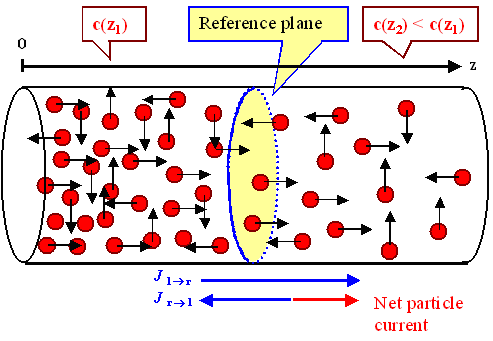Diffusion current through some reference plane.The red spheres symbolize carbon atoms in iron (or phosphorous atoms in silicon, or...); the arrows indicate the next jump they are going to make. Only up/down and left/right jumps are indicated since I can't do better in drawing this. The surface of the iron is on the left, and we assume that there is always plenty of carbon available on the surface but don't show that for clarity's sake.
The little devil looks at a circular reference plane at some depth z. It's circular but only because that makes drawing easier. You are free to imagine any shape you like. The cylinder is meaningless and only there "to guide the eye".
The way I have drawn this figure, we have a decrease in the carbon concentration c(z) going from left to right or with increasing z. That is the reason why on average more carbon atoms will jump through the reference plane from left-to-right (l-r) than from right-to-left (r-l). The little devil would measure a larger partial current jl-r than in the opposite direction. The net current is j = jl-r - jr-lNow that we know what particle currents are, we need to wonder about the reasons for their existence. First thing to note is that there are no particle currents in systems that have achieved nirvana thermodynamic equilibrium. That's easy to see. Wherever a particle current flows, things change. After all, the particle current transports particles from here to there and that means that after a while you have less particles here and more there. As long as something changes, you can't have nirvana or thermodynamic equilibrium as we call it in the science section.It is thus the local particle concentration that changes if there are (net) particle currents. Let's put that in a rule:
Particle currents cause concentrations changes.
You and I have no problem seeing this.It took the genius of Adolf Fick to realize that the deeper truth is to express this the other way around. Cause and effect in the sentence above are related upside down. Turn it around and you haveSo what is a gradient? The difference of something like a concentration c(x) here at the position x and a little bit off at c(x + Dx). Divide by Dx and you have the gradient. In other words, a gradient in one dimension is simply the slope or derivative dc(x)/dx of the c(x) function. The only reason for using a new word is that we need to extend the concept of "slope" to arbitrary dimensions.
If you don't get this you must go back to start. I can't teach you elementary calculus on the side just so. Of course, if you should decide to send me lots of money, I might reconsider.In three dimensions the gradient is written "grad" or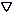(read "napla") and if applied to a function like c(x, y, z) produces a vector with the componentsc(x, y, z)  =  (c(x, y, z)/x,    c(x, y, z)/y,   c(x, y, z)/z)The /x, y, z are simply the partial derivatives of the function, done in the usual way for the particular variable, assuming the others to be constants. Nothing to it. You also see that "havancd math" is to some extent just the invention of smart abbreviations.
The napla operator "" is an extremely powerful abbreviation. It makes equations short and easy to manipulate, saving a lot of writingGood old Adolf Fick figured that not only do concentration gradients drive the particle currents we call diffusion currents, but that these currents are simply proportional to the concentration gradients.Bingo, we have Fick's first law:

j(x, y, z)  =  – D ·c(x, y, z)

spelled out:

jx =  – D · c(x,y,z)
x
jy =  – D · c(x,y,z)
y
jz =  – D · c(x,y,z)
zD is the required proportionality coefficient and called "diffusion coefficient. The "" (minus) sign is convention, it simply makes sure that the mathematical particle current flows in the same direction as the particles. That is not really required. Electrons, for example, flow in opposite direction of the technical electrical current because when the electrical sign conventions were made, nobody had the faintest notion of what was really flowing.Fick's first law states, for example, that there is always a net flow of diffusing particles from the higher to the lower concentration.
Big deal! You would know that without fancy equations! Yes - but now we can calculate what happens! For simple situations and for complicated ones. For carbon diffusing into iron and diffusing out of it. And so on.Before I move on to the more important second law, let me make one thing perfectly clear:
A concentration gradient is always what we call a driving force for particle currents, but it is not the only driving force there is. Electrical potential differences (vulgo called "voltage") drive currents of charged particles, pressure differences drive the flow of gas particles, gravitation drives the flow of water and pretty much everything else, and so on.
Semiconductor electronics, for example, results to a large extent from the competition of the diving forces "potential differences" and "concentration gradients". Both are driving forces that drive currents of charged particles and thus electrical currents inside semiconductor devices, and they tend to drive the charged particle in different directions. The resulting balance determines electrical current flow as a function of voltage and particle concentration and thus the behavior of the deviceFick's first law, in the money analogy, determines how much money flows into or out of your account as a function of money that's somewhere else. If your landlords account, for example, contains less money than there should be, we have a kind of concentration gradient that drives some money from your account into your landlords account.
But now let's move to Fick's second law. It makes a statement on how the balance of your account develops with time or in more general terms how local concentrations change with time.
It is easy to derive this. All we need to do is to balance the account, subtracting everything that flows out in a given time from everything that flows in.
Let's do that in one dimension for starters: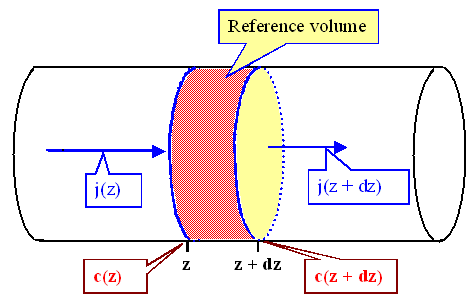Balancing particle flow in some small volumeOnce more, drawing a cylinder is just for convenience. We are looking at the (differentially) small volume between z and z + dz. The change of the volume concentration with time, i.e. dc(z)/dt is simply given by the difference of what flows in,j(z)/dz, minus what flows out, j(z + dz)/dz.Why do I divide the currents by dz? Because we have to convert the areal density of the current (per cm2) into a volume density (per cm3). If 5 people per minute keep flowing into a room with 10 m length, the volume density in there is only half of what it would be in a room with 5 m length. Ponder it, or believe me.
If we write that down we get:

dc(x,t)
dt
=  j(x) – j(x +dx)
dx
=  – dj(x)
dxWe know j at any position from Fick's first law. All we have to do is to substitute j in the equation above by Fick's first law. If we do that (and go to three dimensions right away), we obtain Fick's second law:

¶c  =  D · ¶t æ ç è ö ÷ øD =2 is the delta operator, showing once more that smart abbreviations save a lot of work.Fick's second law simply states that the change in concentration somewhere in your specimen (c(x,y,z/t) in math lingo) that is caused by diffusion is proportional to the second derivative of the concentration there. The proportionality constant once more is the diffusion coefficient D.Of course, whenever we process a piece of material by employing diffusion of something, we need to know how the concentration at some point given by the coordinates x, y, z changes with time t.
The concentration is thus a function of four variables, c = c(x, y, z, t), and Fick's second law is a partial differential equation of second order because it relates partial derivatives (including those of second order) of some unknown function to each other.
Solving partial differential equations for some starting and boundary conditions given by the particular problem you are looking at, is considered an art. Fick's second law is a kind of partial differential equation that is notoriously difficult to solve. I'll give you a short taste treat below.

Using Fick's Second Law for a Standard DiffusionLet's do a simple one-dimensional standard diffusion. We have a piece of iron, put a lot of carbon on the surface with a concentration c0 that is always the same, heat up the whole contraption and watch the carbon diffuse into the iron. In other words, we case harden our blade.Or we do it with phosphorous and a silicon crystal. In this case we need to know what happens with extreme precision because we are now starting to make tiny electronic circuits.
Or we do it with .... It's easy to come up with any number of examples because this is essentially the standard "experiment" concerning diffusion of something into something.We can easily guess on how the concentration profile of the carbon inside the iron, i.e. the concentration as a function of depth, must develop with time. The concentration will be highest close to the surface, decreasing with depth. For longer times, the penetration of the carbon into the depth of the iron will increase. We are going to find some carbon deeper and deeper inside the iron if we keep the experiment running.
We can confidently draw some quantitative diffusion profiles as shown below.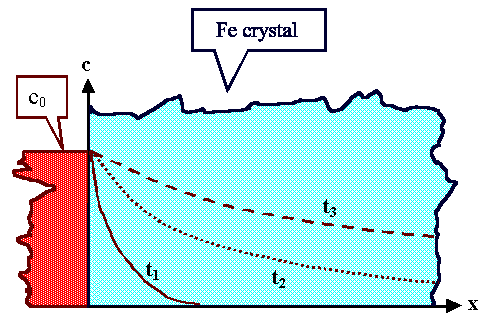Standard diffusion experiment and (guessed) diffusion profiles for increasing diffusion time t3 < t2 < t1.Now let's calculate those diffusion profiles by solving the differential equation called Fick's second law for the peculiarities of our experiment. This peculiarities translate into what is called boundary and starting conditions. We must express them in mathematical terms - here they are.
• Boundary condition: c(x = 0) = c0 = const..
• Starting condition: c(t = 0, x > 0) = 0.It's those conditions that make solving differential equations hard. I spare you the gruesome details and give you the standard solution straight away:

c(x, t)  = x  c0  –  c0 · (D · t)1/2 erf æ ç è ö ÷ ø???? What the f... is an erf? Relax. It is just the harmless error function.
There are just far more functions out there, defined by some name and an abbreviation of that name (e.g. the familiar (?) sine (sin) and cosine (cos)) than you are probably aware of. The error function (erf) belongs to the group of stochastic or statistical functions, just like the more familiar "normal distribution" or "bell curve". There is no abbreviation for the "normal distribution" because we actually can write it out. In fact, the error function is a close relative of the normal distribution, defined by

erf (x) = x ó õ 0Thanks a lot! Well, I can't help it. Solutions of diffusion problems via Fick's laws tend to contain statistical functions - as well they should, considering that the underlying processes are random walks or completely statistical events.
Anyway, the solution given describes exactly the kind of simple curves we came up with by guessing. Except now we have precise numbers.Enough of that. Let's turn to the last problem in the phenomenological description of diffusion. That is, of course, the diffusion coefficient D.
We need a number for that entity if we want to do calculations. What has Adolf Fick done for us in that respect?Nothing at all. The diffusion coefficient for a certain problem like A diffusing in B at the temperature T, i.e. D(A, B, T), is a number that Fick and his contemporaries had to determine from experiments. All they could deduce was that its unit must be [D] = cm2/s.
If you, like Fick, didn't know that things are made from atoms, and that those atoms jump around in a random manner during diffusion, you simply could not take a shot at the diffusion coefficient of a given diffusion problem. As we know now, it must somehow contain what is going on on the fundamental level because that has not been considered so far.Enter Albert Einstein (and a few others like Smoluchowski). They showed (indirectly, and in a way different from what is given below) that the 1. Fick equation can be derived from looking at all this jumping around and that we get an expression for the diffusion coefficient in terms of atoms making jumps.
That will be the topic of the last paragraph.

Connecting Fick's Laws and Jumping AtomsLet's look at a cubic primitive element crystal as shown below. We are going to look a the (1-dim.) net current jV of vacancies moving through some plane P1 at the coordinate x. That means that the same net current of atoms moves in the opposite direction.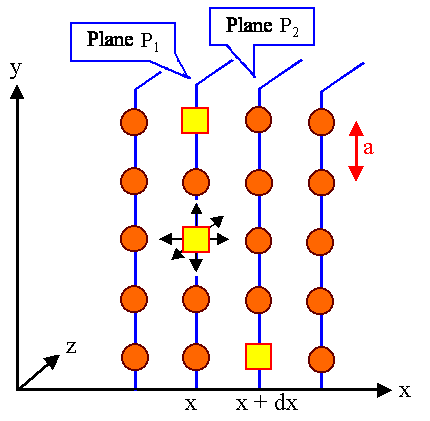Model for the diffusion current of vacancies in a cubic primitive crystal.For doing that, we need to know how many vacancies Pi per cm2 are contained in the various planes Pi. In other words, we need to know the areal density and not the volume density cV(x). We also allow that this density changes with x, i.e. that we have a density gradient.
For getting areal densities out of volume densities one multiplies cV(x) with the lattice constant a. We have

P1,V  =   a · cV(x)

P2,V  =   a · cV(x + dx)  = a · cV(x + a)I have replaced an infinitely small dx by a small but finite lattice constant a. Real mathematicians will recoil in horror from am atrocity like this and will stop speaking to you - but you don't want to talk to these guys anyway. In the world of crystals, dimensions smaller than about a lattice constant simply make no sense, so we are perfectly justified in doing this.
Now let's consider the jumping around of the vacancies in the the two neighboring planes P1 and P2. We are only interested in jumps in the x-direction since all the others do not contribute to the particle movement in this direction. The jumping rate we call r once more. Looking at it in detail gives us
• r1-2 = jumping rate in +x direction from P1 to P2 = 1/6 · r(x)
• r2-1 = jumping rate in x direction from P2 to P1 = 1/6 · r(x +a)Since in our geometry only 1 out of 6 possible jumping directions points in the desired direction (look at the figure to appreciate this), we take only 1/6 of the total number of jumps per second. In different types of crystal, this lattice factor g is somewhat different. For fcc or bcc crystals we have g = 1. It is not all that difficult to figure that out for yourself. Otherwise just believe me.
The diffusion current of vacancies in one direction is simply given by how many vacancies will jump through the area per second, and that is the jumping rate times the number of vacancies per area that we have figured out above. We have, for example:
j1-2  = r · a · c(x) / 6
The net diffusion current jx of vacancies diffusing in x-direction then is j1-2j2-1. Putting it all together (in reverse order and thus with reversed signs) we have

jx =   j1-2  –   j2-1  =  – (j2-1  –   j1-2)

jx = a · r   · – 6 æ è ö ø ÷ ÷Expanding the expression by a/dx, once more using a or dx as essentially the same thing, gives the final result

jx  =  –  a2 · r
6
·   c(x + dx)  –  c(x)
dx
=  –   a2 · r
6
·  dc(x)
dxBingo once more! That is Fick's second law for one direction. Except that we have (a2 · r / 6) instead of the diffusion coefficient D. So that expression is the diffusion coefficient:

D(T)  =  g · a2 · r  =  g · a2 · n · exp–(ED/kT)

D(T)  =  D0 · exp–(ED/kT)

g = lattice factor, ED = diffusion energy of the diffusing particle,
D0 = pre-exponential factor of diffusion coefficient, and
r = n · exp–(ED/kT)This is a pretty momentous equation. Let's savor it bit by bit.1. The "phenomenological" diffusion coefficient in Fick's laws is now fully explained. It is essentially the jumping rate r(T) of the diffusing particle that is doing a random walk. For vacancy assisted diffusion, this is the jumping rate of the vacancy, for interstitial diffusion it is the jumping rate of the interstitial. Of course, the direction of the diffusion current of the atom diffusing by a vacancy mechanism is opposite to that of the vacancy current.
The jumping rate must be multiplied by the lattice constant a, to take care of dimensions, and the lattice factor g, to describes the different geometries in different types of crystals. For a simple element crystals (one kind of atoms) we have g = 1/6, 1, 1, 1/8 for cubic primitive, fcc, bcc or diamond type, respectively.
There is more. The diffusion coefficient contains:
• The number of steps N that a randomly walking particle has done in 1 second, which is simple the jumping rate r(T) times time t or
N = t · r(T).
• The step length, which is the lattice constant, give or take a factor like 2½ to adjust for the detailed geometry.
We thus can express our old friend the diffusion length L as

L  =  a · (N)½      "Old" equation

L  =  (D · t )½      New equation

In words: The diffusion length, a kind of average penetration depth of A diffusing into B, scales with the square root of the time t and the square root of the diffusion coefficient D. If you want your carbon to penetrate 10 times as deep into your iron, you must hold it 100 times longer at some high temperature.2. Since "thermally activated" diffusion, or jumping of particles over an energy barrier, always contains a Boltzmann factor and thus an exponential dependence on temperature, the diffusion coefficient changes dramatically with temperature. It thus does not make sense to give a number for it. What's needed are at least two numbers (ED and D0) or a graph, always in the Arrhenius plot as we know it from looking at vacancy concentrations.3. The diffusion energy ED for a jump of a vacancy is not always the same number as EM, the energy that an atom of the crystal needs to overcome in order to jump into a neighboring vacancy. We must expect it to be different if some foreign atom sitting in the lattice does the jump. If an interstitial jumps, EM has nothing to do with EM anyway.4. Besides the Boltzmann factor, we only have a bunch of constants (there are a few more and rather unimportant ones if one looks at the situation a bit more closely) like the vibration frequency n and lattice parameters. We simply merge all that to the "pre-exponential" factor D0.5. Not to forget, Fick's first law is now a law of nature. It is valid in all cases where the basic ingredients (jumping at random etc.) are given. For other cases, we know (in principle) what we need to do.
Fick's second law follows automatically from Fick's first law if we assume "continuity". That simply means that our diffusing particles can neither be destroyed nor created. They do not suddenly disappear into nothing or appear out of nothing. That is certainly true for common atoms but not, for example, for particles like neutrons that decay radioactively into something else, or photons that get absorbed and generated all the time. In this case Fick's second law must be augmented, and it is perfectly clear how to do that.5. Just to be on the save side. Einstein didn't really do it as shown above. His reasoning - big surprise! - was far more abstract and mathematical. In essence, he explained so-called "Brownian motion"; which leads straight to "random walk theory", which leads straight to what I have shown above.Let's sum up! All of the above simply means that for any diffusion problem of some A running around in B via a a random walk, we need to know just two numbers:
1. The migration energy ED, and
2. The pre-exponential factor D0
Rather involved calculations give us some idea about the approximate values of these numbers, but so far we need to measure them experimentally if we want to have precise values.The rest "simply" involves solving Fick's law with the numbers provided for the diffusion coefficient D. I'm not saying it is easy. But I am saying that we know how it works.Let's look at a few examples: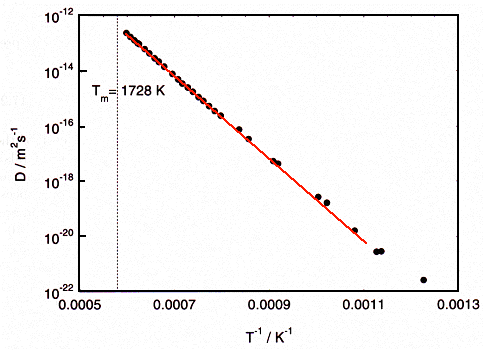Nickel (Ni) self diffusion Source: Helmut Mehrer, "Diffusion in Solids", Springer, 2007These are measured data of the self-diffusion in nickel, a magnetic material. The red line is a perfect straight line and serves only to guide the eye Note that the measured points follow it rather closely. The two numbers for the migration energy and the pre-exponential factor can be extracted easily and are given in the table below.
By the way, Helmut Mehrer, the author of the source book I used a lot here, was the teaching assistant who way back around 1972 tried to teach me about point defects and diffusion. His book is presently the "bible" for everything concerning diffusion in solids. Of course, the data I took from his book were not all measured personally by him but represent the efforts of a large number of scientists.

Fcc metals Cu Ag Au Ni Pd Pt Pb
EM [eV] 2.95 1.77 1.71 2.92 2.77 2.67 1.11
D0 [10-5 m2s-1] 7.8 0.41 0.27 13.3 0.205 0.5 8.87
Self-diffusion dataYou noticed that I did not include data on iron or other bcc metals. There is a reason for this, just bear with me (or jump to the iron module right away). Before I finish this module, let me show you a wealth of diffusion data in a material where it really matters: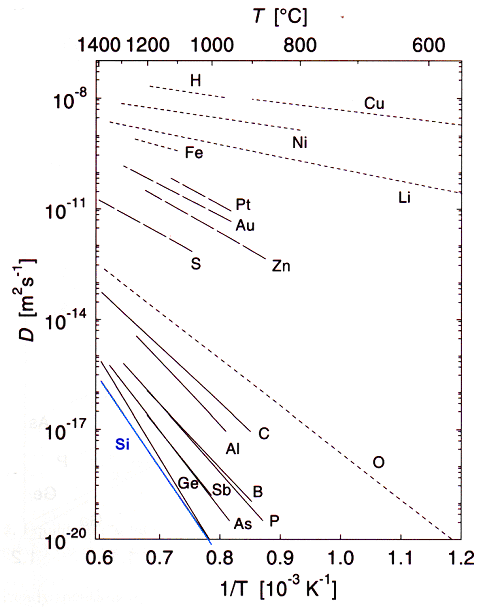Diffusion in silicon (Si) including self-diffusion Source: Helmut Mehrer, "Diffusion in Solids", Springer, 2007What you see, for example, is that the elements of supreme importance to silicon technology (boron (B), arsenic (As) and phosphorous (P)) are diffusing far more slowly than metals like nickel (Ni) copper (Cu ) or iron (Fe). That is too bad because we need to diffuse the first three into the silicon and we must keep everything else out at all costs.
You also see that self-diffusion, the running around of silicon atoms inside the silicon crystal, is the slowest diffusion of all.
And of course you realize that those curves, measured and re-measured many times, show the results of many tricky, expensive and time consuming experiments that had been made over the years.
So let's now look at how you actually do the experiments.Back toDiffusion1. Atomic Mechanisms of Diffusion2. Random WalkOn to4. Experimental Techniques for Measuring Diffusion Parameters5. Diffusion in IronSteel GuysDiffusion in IronBoltzmann DistributionBravais Lattices and CrystalsDiffusion in IronTTT Diagrams: 1. The Basic IdeaOstwald Ripening10.5.2 Making Steel up to 1870Units of Length, Area, and VolumeExperimental Techniques for Measuring Diffusion ParametersPhenomenological Modelling of DiffusionSize and Density of PrecipitatesAtomic Mechanisms of DiffusionRandom WalkMaking Vacancies1. Basics of SegregationSegregation at Room TemperatureGlobal and Local Equilibrium for Point DefectsConstitutional Supercooling and Interface Stability

© H. Föll (Iron, Steel and Swords script)